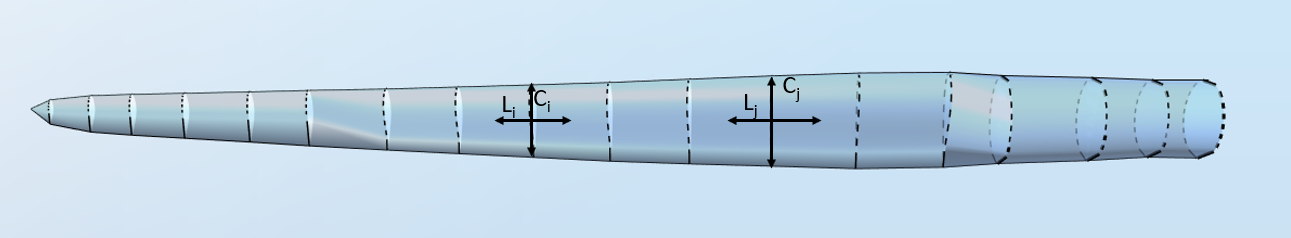# Aspect ratio

The aspect ratio is a geometrical property of the blade. In Ashes, the aspect ratio is only used when applying the Viterna extrapolation Viterna et al. (1982)  to obtain blade-dependent aerodynamic coefficients (i.e. lift, drag and moment coefficient) for angles of attack ranging from -180 to 180 degrees.

In Ashes, the aspect ratio is defined as
$$AR = \frac{(B_L)^2}{A_{pf}}$$

where
• $$B_L$$
• $$A_{pf}$$
is the planform area
The planform area is the sum, for each blade element, of the product of the Chord length times the influence length. It can thus be defined as
$$A_{pf}=\sum_i^{elts}C_i\cdot L_i$$

where
The image below shows an example of the chord length and the influence length of two aerodynamical stations of the NREL 5 MW blade.Note:
• the influence length of an aerodynamical station is sum of half the distances to the (two) stations on either side of the station in question.
• the chord length is given in the Blade shape file. The twist angle is not considered in the definition of the planform area

By summing up the product of the chord length and the element length for all elements in the NREL 5 MW blade, the following planform area, highlighted in red, is obtained:Other definitions of the aspect ratio can be found in the literature. For example, Tangler et al. (2004b) define the aspect ratio as the ratio between the blade length and the chord length at 80% of the blade length, starting from the root.